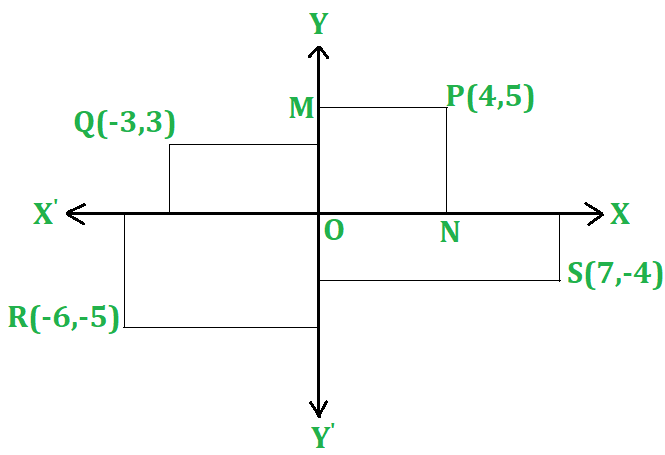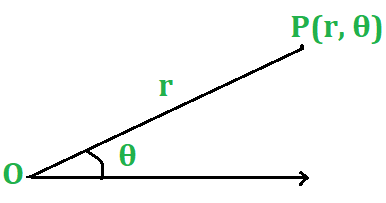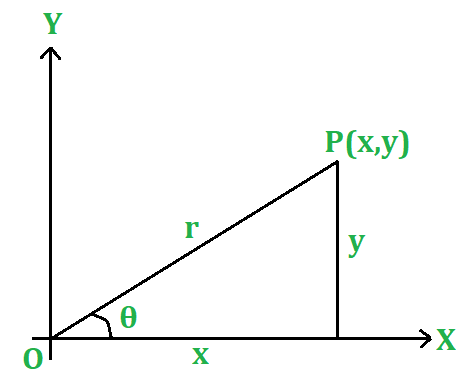# Coordinate Geometry

• Last Updated : 11 Jun, 2021

Co-ordinate geometry or analytical geometry is the branch of geometry in which algebraic equations are used to denote points, lines, and curves. Coordinate Geometry is the unification of algebra and geometry in which algebra is used in the study of geometrical relations and geometrical figures are represented by means of equations. The most popular coordinate system is the rectangular Cartesian system. Coordinates of a point are the real variables associated in an order to describe its location in space. Here we consider the space to be two-dimensional. Let’s have a look at the following terms.

• Origin: Through a point O, referred to as the origin, we take two mutually perpendicular lines XOX’ and YOY’, and call them x and y axes respectively.
• Abscissa & Ordinate: The position of a point is completely determined with reference to these axes by means of an ordered pair of real numbers (x, y) called the coordinates of P where | x | and | y | are the distances of the point P from the y-axis and the x-axis respectively, x is called the x-coordinate or the abscissa of P and y is called the y-coordinate or the ordinate of the point P.Attention reader! All those who say programming isn't for kids, just haven't met the right mentors yet. Join the  Demo Class for First Step to Coding Coursespecifically designed for students of class 8 to 12.

The students will get to learn more about the world of programming in these free classes which will definitely help them in making a wise career choice in the future.

Let P be a point in a plane; draw two perpendicular lines X’OX, Y’OY in the plane, and draw PM, PN parallel to OX, OY respectively. X’OX, Y’OY is known as the coordinate axes. MP is the x-coordinate, called the abscissa, and NP is the y-coordinate called the ordinate of P.

### Polar Coordinates

Let OX be a given line and P be any point in a given plane. Let 0 be the angle through which a line rotates in moving from OX to the position OP. Then, if r is the length of OP, the position of P is known when r and θ are known. r is called the radius vector, the vectorial angle, and (r, θ) is the polar coordinates of the point P, which is briefly referred to as the point (r, θ). OX is called the initial line and  O the pole.### The Relation Between Cartesian and Polar Coordinates

Let P be the point (x, y) with reference to rectangular axes OX, OY, and the point (r, θ) with reference to the pole O and initial line OX. i.e., the X-axis. We have x = rcosθ; y = rsinθ Hence tanθ = y/x and x2 + y2 = r2Х These relations enable us to change from one system of coordinates to the other.## Distance Between Two Points

The distance between two points A(x1, y1) and B(x2, y2) is given by

AB = √((x2 – x1)2 + (y2 – y1)2)

The distance of the point P(x, y) from the origin O(0, 0) is given

OP = √((x – 0)2+ (y – 0)2), i.e. OP = √(x² + y²)

### Examples

Example 1: Find the distance between the points P(-4, 7) and Q(2, -5)?

Solution:

The given points are P(-4, 7) and Q(2, -5).

Then, (x1 = -4, y1 = 7) and (x2 = 2, y2 = -5). :

PQ =  √((x2 – x1)2 + (y2 – y1)2)

= √((2 – (-4))2 + (-5 – 7)2)

= √(62 + (-12)2

= √(36 + 144)

= √180

= 6√5

Example 2: Find the distance of the point P(6, -6) from the origin?

Solution:

Let P(6, -6) be the given point and O(0, 0) be the origin.

Then, OP = √((6 – 0)2 + (-6 – 0)2)

= √(62 + (-6)2)

= √72

= 6√2

## Section Formula

The coordinates of the point P(x, y) which divides the line segment joining A(x1, y1) and B(x2, y2) internally in the ratio m:n are given by

x = (mx2 + nx1) / (m+n)

y = (my2+ny1) / (m+n)

### Examples

Example 1: Find the coordinates of the midpoint of the line segment joining the points A(-5, 4) and B(7,-8)?

Solution:

Let M(x, y) be the midpoint of AB. Then,

x = ((-5) + 7)/2 =1 and  y = ((4 + (-8)) = -2

Hence, the required point is M (1, -2).

Example 2: Find the coordinates of the points of trisection of the line segment joining the points A(-5, 6) and B(4, -3)?

Solution:

Let P and Q be the points of trisection of AB.

Then, P divides AB in the ratio 1 : 2

So, the coordinates of P are

P((1 x 4 + 2 x (-5)/1 + 2, ((1 x (-3) + 2 x 6)/1 + 2) i.e., P(-2, 3).

Also, Q divides AB in the ratio 2:1.

So, the coordinates of Q are

Q((2 x 4 + 1 x (-5)/2 + 1, (2x(-3) +1 x 6/1 + 2)) i.e., Q(1, 0)

Q(1, 0).

## Area of Triangle

The area of a triangle ABC with vertices A(x1, y1), B(x2, y2), and C(x3, y3) is given by

area(ABC) = |1/2 {x1(y2 – y3) + x2(y3 – y1) + x3 (y1 – y2)}|

### Examples

Example 1: Find the area of the triangle whose vertices are A(2, 7), B(3, -1) and C(-5, 6)?

Solution:

Let A(2, 7), B(3, -1) and C(-5, 6) be the vertices of the given △ABC.

Then,

(x1 = 2, y1 = 7), (x2 = 3, y2 = -1) and (x3 = -5, y3 = 6).

Area of △ABC = 1/2|{x1(y2 – y3) + x2(y3 – y1) + x3(y1 – y2)}|

= 1/2 |2(-1 – 6) + 3(6 – 7) – 5(7 +1)|

= 1/2| -14 – 3 – 40|

= 1/2|-57|

= 57/2

= 28.5 sq units.

Example 2: Find the area of quadrilateral ABCD whose vertices are A(-4, -2), B(-3, -5), C(3, -2), and D(2, 3).

Solution:

Join A and C. Then, area of quad. ABCD = ar(△ABC) + ar(△ACD)

Area of  △ABC = 1/2|{(-4).(-5 + 2) -3(-2 + 2) + 3(-2 + 5)}|

= 21/2 sq units.

Area of  △ACD = 1/2|{ (-4).(-2 – 3) + 3(3 + 2) + 2(-2 + 2}|

= 35/2

Area of quad. ABCD = 21/2 + 35/2  sq units = 28 sq units.

## Condition for Collinearity of Three Points

Let the given points be A(x1, y1), B(x2, y2), and C(x3, y3). Then A, B, and C are collinear,

⇒ area of ABC = 0

⇒ 1/2.[x1(y2 – y1) + x2(y3 – y1) + x3(y1 – y2)] = 0

### Examples

Example 1: Show that the points A(-1, 1), B(5, 7), and C(8,10) are collinear.

Solution:

Let A(-1, 1), B(5, 7) and C(8, 10) be the given points.

Then, (x1 = -1, y = 1), (x2 = 5, y2 = 7) and (x3 = 8, y3 = 10)

∴  x1(y2 – y3) + x2(y3 – y1) + x3(y1 – y2)

= (-1) (7 – 10) + 5(10 -1) + 8(1 – 7)

= (3 + 45 – 48)

= 0

Hence, the given points are collinear.

Example 2: Show that the points A(a, b + c), B(b, c + a) and C(c, a + b) are collinear.

Solution:

Let A(a, b + c), B(b, c + a) and C(c, a + b) be the given points.

Then, (x1 = a, y1 = b + c); (x2 = b, y2 = c + a); and (x3 = c, y3 = a + b).

∴ a(c + a – a – b) + b(a + b – b – c) + c(b + c – c – a)

= a(c – b) + b(a – c)+ c(b – a)

= 0

Hence, the given points are collinear.

My Personal Notes arrow_drop_up